# B-spline Curves: Inserting a Knot Multiple TimesIn many applications, a knot is required to be inserted multiple times. A simple method for inserting the same knot multiple times is to repeatedly apply the knot insertion algorithm. However, in doing so, the original knot vector and the original set of control points must be modified after each insertion, which is a rather tedious task. Fortunately, by studying the behavior of the knot insertion algorithm, we can find a much simpler way to do the same.### Observation I: The Coefficients for Computing the New Control Points

Let us start with a simple case. Let t be inserted in the middle of knot span [uk, uk+1) and let the degree be p. The coefficients ai,1's for the first insertion, where k-p+1 <= i <= k, are computed as follows: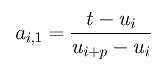After t is inserted, it becomes a knot and knots uk+1, uk+2, ..., um will be shifted one position to the right. More precisely, the new knots, v0, v1, ..., vk, vk+1, ..., vm and vm+1 are

 First Insertion v0 v1 ..... vk vk+1 = t vk+2 ..... vm vm+1 Original u0 u1 ..... uk t uk+1 ..... um-1 um

Note that vk+1 divides the new knot vector into two halves. Knots to the left of vk+1 = t are identical to the corresponding values of the original, while knots to the right of t satisfy the relation vh = uh-1.

If t is inserted again, it lies in knot span [vk+1,vk+2). Since t is equal to the left end, this second insertion makes vk+1 a double knot. The coefficients ai,2's for this second insertion, based on the new knot sequence, are computed as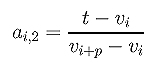where i is less than or equal to k+1. Rewriting the above using u we have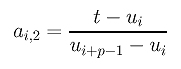Note that the ui's are on the left-hand side of t = vk+1 and the ui+p's are on the right-hand side of v = vk+1.

Let this new set of knots be wi's. The relation of this new set and the origin is shown below:

 Second Insertion w0 w1 ..... wk wk+1 = wk+2 = t wk+3 ..... wm+1 wm+2 First Insertion v0 v1 ..... vk vk+1 = t vk+2 ..... vm vm+1 Original u0 u1 ..... uk t uk+1 ..... um-1 um

Let t be inserted a third time, which makes wk+1 a triple knot. It is not difficult to see that the coefficients ai,3's for this third insertion are computed as follows: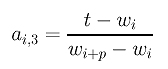The above can be rewritten as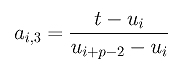Generalizing this idea, ai,h's, the coefficients for the h-th insertion, are computed as follows: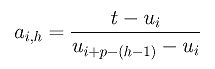What if in the original knot vector uk is a multiple knot with multiplicity s and t is inserted at uk? Would this affect the above formula? Fortunately, nothing will change. Let us see why. If uk is a multiple knot of multiplicity s, then uk = uk-1 = uk-2 = ... = uk-s+1:

 w0 w1 ..... wk-s+1 = ... = wk-2 = wk-1 = wk = wk+1 = wk+2 = t wk+3 ..... wm+1 wm+2 v0 v1 ..... vk-s+1 = ... = vk-2 = vk-1 = vk = vk+1 = t vk+2 ..... vm vm+1 u0 u1 ..... uk-s+1 = ... = uk-2 = uk-1 = uk = t uk+1 ..... um-1 um

Because all of them lie to the left-hand side of t and because the shifting of indices only happen to those knots to right of t, the computation formula for ai,h does not have to be modified. Therefore, we conclude that

 If a new knot t is inserted to knot span [uk, uk+1) h times, the coefficients ai,h can be computed as follows:### Observation II: The Computation of New Control Points

Recall from the way of inserting a knot at an existing knot that if t is inserted at an existing knot uk of multiplicity s, then control points Pk-s, Pk-s+1, ..., Pk-1 and Pk are not affected and only Pk-p, Pk-p+1, ..., Pk-s participate in the computation of new control points Qi's. Let us rewrite all new control points after the first insertion with a second subscript 1. Thus, we have the following diagram: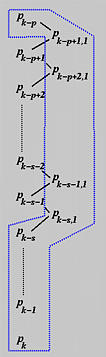If the same knot is inserted the second time, the affected p+1 control points are Pk, Pk-1, Pk-s, Pk-s,1, Pk-s-1,1, ..., Pk-p+1,1 as shown in the above diagram. Note that Pk-p is excluded because only p+1 control points are affected. However, since the multiplicity of t is increased by one due to the previous insertion, s+2 control points will not participate in the knot insertion computation: Pk, Pk-1, ..., Pk-s and Pk-s,1. The computation scheme and the new set of control points are shown below. Note that new control points generated from the second insertion has 2 as the second subscript. The new control points are: (1) Pk-p+1,1 and Pk-s,1 computed from the first insertion, and (2) Pk-p+2,2 to Pk-s,2 computed from the second insertion.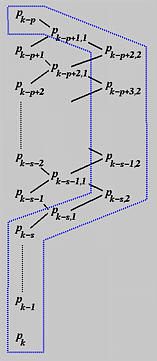For the third insertion, the affected p+1 control points are Pk-p+2,2 to Pk-s,2, Pk-s,1, and Pk-s to Pk. Since this third insertion increases the multiplicity of t by one again, the number of control points that will not involve in the computation is also increased by one. As a result, Pk-s,2 will be the same before and after the third insertion. This is the meaning of the following diagram: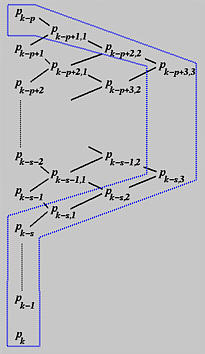In general, if t is inserted h times at a knots uk of multiplicity s, where s = 0 means t is inserted in the middle of that knot span and s > 0 means t is inserted at a knot uk of multiplicity s, one can

1. write down the first set of p+1 affected control points as the 0-th column;
2. ignore the last s control points (i.e., Pk-s+1 to Pk);
3. compute the first column, the second column, ... and the h-th column;
4. the new set of control points are those surrounded by the blue polygon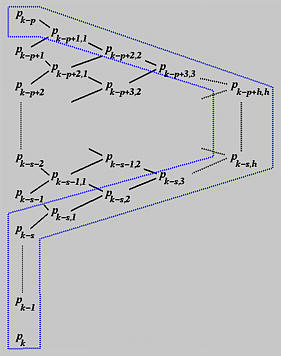### A Summary

Combining these two observations, we have the following algorithm for inserting a knot t multiple times.

Input: knot t in [uk, uk+1) with initial multiplicity s to be inserted h times, where h+s is less than or equal to p, the degree of the given B-spline curve
Output: a new set of control points after t is inserted h times

Let control points Pk, Pk-1, ..., Pk-p be renamed as Pk,0, Pk-1,0, ..., Pk-p,0 by adding a second subscript 0.

for r := 1 to h do
for i := k-p+r to k-s do
begin
Let ai,r = (t - ui) / ( ui+p-r+1 - ui )
Let Pi,r = (1 - ai,r) Pi-1,r-1 + ai,r Pi,r-1
end

The new set of control points are constructed from the original ones from P0 to Pk-p, followed by the top edge of the diagram above (i.e., Pk-p+1,1, Pk-p+2,2, ..., Pk-p+h,h), followed by the right edge of the diagram (i.e., Pk-p+h+1,h, Pk-p+h+2,h, ...., Pk-s,h), followed by the bottom edge of the diagram (i.e., Pk-s,h-1, Pk-s,h-2, ...., Pk-s,1), followed by the original control points Pk-s, ..., Pk, ..., Pn.### Corner Cutting

As mentioned earlier, knot insertion is a corner cutting process. For example, suppose we have a B-spline curve of degree 6 and want to insert u twice at a knot u10 of multiplicity 2. The affected control points are P10, P9, ..., P4, since k = 10, p = 6 and k-p = 4. Since the multiplicity of u10 is 2, we have s = 2 and u10 and P9 are not changed. The first insertion produces P8,1, P7,1, P6,1 and P5,1 and the corners at P7, P6 and P5 are cut. The new set of control points contains P0 to P4, P5,1, P6,1, P7,1, P8,1, P8, P9, P10, .....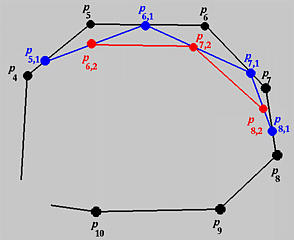The second insertion produces P8,2, P7,2 and P6,2. Thus, the corners at P7,1 and P6,1 are cut and the new set of control points are P0 to P4, P5,1, P6,2, P7,2, P8,2, P8,1, P8, P9, P10, .....